HomeFee ScheduleCalc PagesCurriculum Vitae -->

 Introduction Crush Calculator Supporting Physics FormulasIntroduction

One way in which Accident Reconstructionists are able to estimate the speed of a vehicle is through the process of Crush Analysis. In this analysis, the damage displacement is measured in inches at equal distances from each other across the damage area. These measurements are referred to as “C” measurements and are listed in order C1, C2, C3, C4, etc. The width of the contact damage area is also measured in inches. Additionally, the angle of force (PDOF) in degrees is determined by analyzing the contact damage area and determining the direction in which the components have been displaced.

Additional information required for the calculation involves the stiffness values and weight of the vehicle. The “A” value used in the calculations refers to the maximum force per inch that will not cause permanent damage. The “B” value refers to the spring stiffness per inch of damage width. The “G” value is calculated by placing the “A” and “B” values into the formula A^2/2B. The “A” and “B” values can be obtained from crash test data from NHTSA and other organizations. For example, a Buick LeSabre has A and B stiffness values for the front surface of the vehicle of 356 and 34, respectively. A Pontiac Firebird has A and B stiffness values of 317 and 56, respectively. The weight of the vehicle refers to the gross weight including cargo and passengers. The weight of the vehicle can be determined through published data or by weighing the vehicle.

The Crush Calculator determines the closing velocity of two vehicles based on the amount of damage sustained during impact. All measurements are entered in inches and degrees.

Crush Calculator

This program requires Java 2 to run

Supporting Physics

Although many of the laws of physics are used in Accident Reconstruction, the two laws primarily used in Crush Analysis are Hooke’s Law and the law of Conservation of Energy. Hooke’s Law states that the amount of force required to compress a spring is directly proportionate to the distance that the spring is compressed. Hooke’s law, which was developed by Robert Hooke several hundred years ago, also applies to the amount of force required to crush a vehicle. The law of Conservation of Energy states that energy is neither created nor lost. Therefore, when an event occurs such as a collision between two vehicles, the total amount of energy that was present before the collision is equal to the amount of energy after the collision. The energy present before the collision is the kinetic energy of the moving vehicle(s). During the event, one way that the energy changes form is the damage done to the vehicles. Using these principles, the pre-impact speeds of the vehicles can be determined.

For more information on this topic, refer to SAE Technical Paper 2000-01-1318 "Derivation of Closing Speed as a Function of Dissipated Energy" © 2000 SAE International written by Edward L. Robinson. Click here to view the paper.

Energy from crush in pounds per square inch

 E = Energy in inch pounds that caused the damage W = Width of the damaged area C1 - C6 = Damage displacement measured in inches A, B, G = Stiffness coefficients Ang = Angle of the force that caused the damage (PDOF)

2 C measurements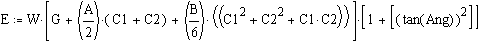4 C measurements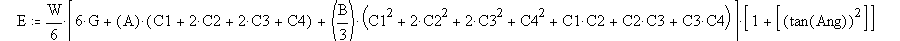6 C measurements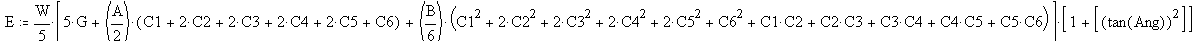Formula for determining Barrier Equivalent Velocity (BEV) in Feet per Second

 BEV = Barrier Equivalent Velocity in Feet Per Second Ef = Energy in Foot Pounds w = the weight of the vehicle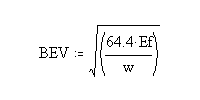Formula for determining Closing Speed of the Two Vehicles

 Vcl = The closing speed in Miles per Hour E = The total energy in foot pounds g = Gravitational constant (32.2) Wa = Weight of Va Wb = Weight of Vb e = Coefficient of restitution (the amount of "bounce back" of the damage)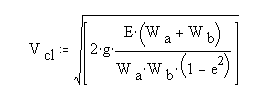<<

Text and applet are Copyright 2004 Daniel Kender